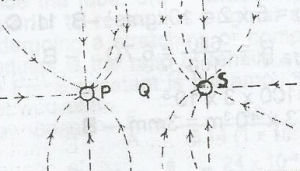The diagram above shows the resultant electric field pattern due to two electric point charges P and S. Which of the following statements is correct?

A. P is negatively charged while S is positively charged
B. P is positively charged while S is negatively charged
C. both P and S are positively charged
D. both P and Q are negatively charged